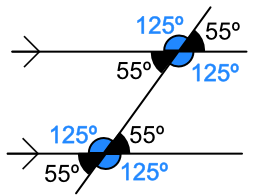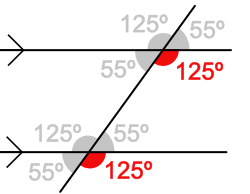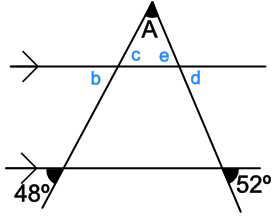Angles and Parallel Lines

# Angles and Parallel Lines

GCSE(F),

When Parallel lines are crossed by another line, a number of angles are created between the lines. Parallel lines are shown with arrows. The first pair of parallel lines on a drawing is shown with a single arrow; the second pair with a double arrow, and so on.When a line crosses a pair of parallel lines, it creates two sets of angles. Each set of angles has the same value. Note that each adjacent pair of angles add up to 180º, because they are a straight line.Angles which are within the parallel lines, but on opposite (or alternate) sides of the single line, are Alternate Angles:Note that, in the above diagram, the pair of 125º angles are also alternate angles.

Angles which appear on the same place on each of the parallel lines are called Corresponding Angles:In the above diagram, there are other pairs of corresponding angles.

## Examples

1. What is the size of the angle marked A?